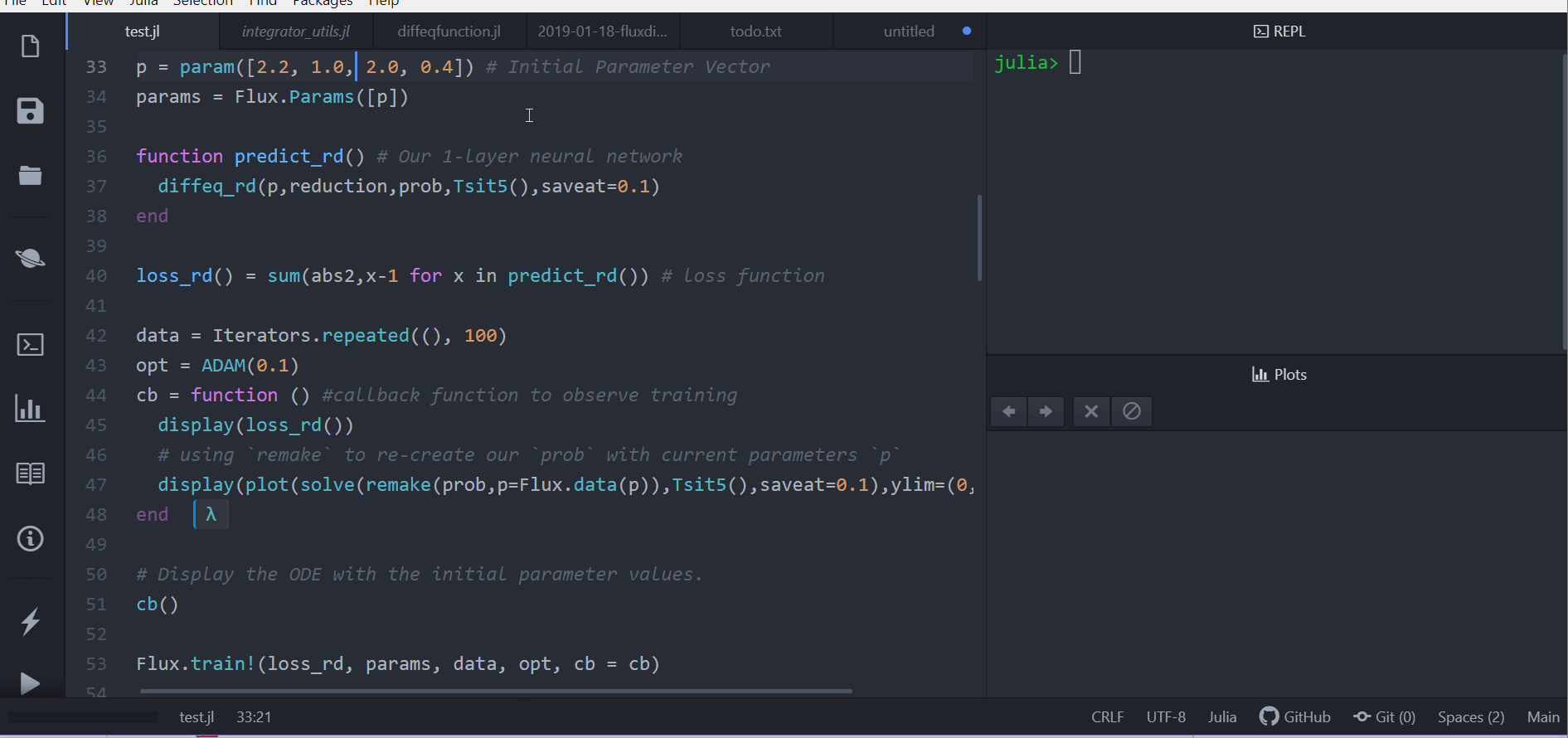# Neural Ordinary Differential Equations with Flux

All of the tools of SciMLSensitivity.jl can be used with Flux.jl. A lot of the examples have been written to use FastChain and sciml_train, but in all cases this can be changed to the Chain and Flux.train! workflow.

## Using Flux Chain neural networks with Flux.train!

This should work almost automatically by using solve. Here is an example of optimizing u0 and p.

using OrdinaryDiffEq, SciMLSensitivity, Flux, Plots

u0 = Float32[2.; 0.]
datasize = 30
tspan = (0.0f0,1.5f0)

function trueODEfunc(du,u,p,t)
true_A = [-0.1 2.0; -2.0 -0.1]
du .= ((u.^3)'true_A)'
end
t = range(tspan,tspan,length=datasize)
prob = ODEProblem(trueODEfunc,u0,tspan)
ode_data = Array(solve(prob,Tsit5(),saveat=t))

dudt2 = Flux.Chain(x -> x.^3,
Flux.Dense(2,50,tanh),
Flux.Dense(50,2))
p,re = Flux.destructure(dudt2) # use this p as the initial condition!
dudt(u,p,t) = re(p)(u) # need to restrcture for backprop!
prob = ODEProblem(dudt,u0,tspan)

function predict_n_ode()
Array(solve(prob,Tsit5(),u0=u0,p=p,saveat=t))
end

function loss_n_ode()
pred = predict_n_ode()
loss = sum(abs2,ode_data .- pred)
loss
end

loss_n_ode() # n_ode.p stores the initial parameters of the neural ODE

callback = function (;doplot=false) #callback function to observe training
pred = predict_n_ode()
display(sum(abs2,ode_data .- pred))
# plot current prediction against data
pl = scatter(t,ode_data[1,:],label="data")
scatter!(pl,t,pred[1,:],label="prediction")
display(plot(pl))
return false
end

# Display the ODE with the initial parameter values.
callback()

data = Iterators.repeated((), 1000)
res1 = Flux.train!(loss_n_ode, Flux.params(u0,p), data, ADAM(0.05), cb = callback)

callback()

## Using Flux Chain neural networks with Optimization.jl

Flux neural networks can be used with Optimization.jl by using the Flux.destructure function. In this case, if dudt is a Flux chain, then:

p,re = Flux.destructure(chain)

returns p which is the vector of parameters for the chain and re which is a function re(p) that reconstructs the neural network with new parameters p. Using this function we can thus build our neural differential equations in an explicit parameter style.

Let's use this to build and train a neural ODE from scratch. In this example we will optimize both the neural network parameters p and the input initial condition u0. Notice that Optimization.jl works on a vector input, so we have to concatenate u0 and p and then in the loss function split to the pieces.

using Flux, OrdinaryDiffEq, SciMLSensitivity, Optimization, OptimizationOptimisers, OptimizationOptimJL, Plots

u0 = Float32[2.; 0.]
datasize = 30
tspan = (0.0f0,1.5f0)

function trueODEfunc(du,u,p,t)
true_A = [-0.1 2.0; -2.0 -0.1]
du .= ((u.^3)'true_A)'
end
t = range(tspan,tspan,length=datasize)
prob = ODEProblem(trueODEfunc,u0,tspan)
ode_data = Array(solve(prob,Tsit5(),saveat=t))

dudt2 = Flux.Chain(x -> x.^3,
Flux.Dense(2,50,tanh),
Flux.Dense(50,2))
p,re = Flux.destructure(dudt2) # use this p as the initial condition!
dudt(u,p,t) = re(p)(u) # need to restrcture for backprop!
prob = ODEProblem(dudt,u0,tspan)

θ = [u0;p] # the parameter vector to optimize

function predict_n_ode(θ)
Array(solve(prob,Tsit5(),u0=θ[1:2],p=θ[3:end],saveat=t))
end

function loss_n_ode(θ)
pred = predict_n_ode(θ)
loss = sum(abs2,ode_data .- pred)
loss,pred
end

loss_n_ode(θ)

callback = function (θ,l,pred;doplot=false) #callback function to observe training
display(l)
# plot current prediction against data
pl = scatter(t,ode_data[1,:],label="data")
scatter!(pl,t,pred[1,:],label="prediction")
display(plot(pl))
return false
end

# Display the ODE with the initial parameter values.
callback(θ,loss_n_ode(θ)...)

# use Optimization.jl to solve the problem

optprob = Optimization.OptimizationProblem(optf, θ)

result_neuralode = Optimization.solve(optprob,
allow_f_increases = false)
Notice that the advantage of this format is that we can use Optim's optimizers, like LBFGS with a full Chain object for all of Flux's neural networks, like convolutional neural networks.